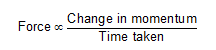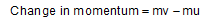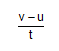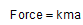# How to derive equation for newtons second law of motion?

How to derive equation for newtons second law of motion?

Newton’s second law of motion states that, “The rate of change of momentum of a body is directly proportional to the applied force and takes place in the direction in which force acts”.Now, momentum is the product of mass and velocity.
Thus, change in momentum isThus, we haveBut,is the acceleration of the body
Thus,?k is the constant of proportionality and in SI units, its value is 1.
F = ma
This is the equation of Newton’s second law of motion.

1 Like